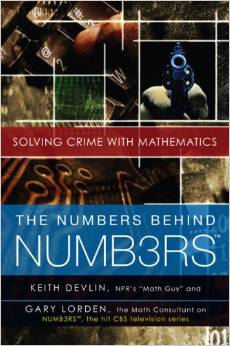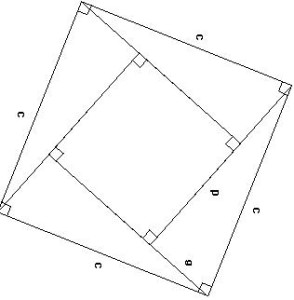## Book Review: The Numbers Behind NUMB3RS: Solving Crime with MathematicsI started watching Numb3rs after reading this book. Actually the book helped appreciating the show since the show itself does not clarify many of the techniques they use and most of the time we just have to trust the show. In that sense this book acts as a companion book to the show itself. But it does more than that. The chapter on network analysis was particularly interesting and the discussion on misplaced degrees of separation a good one. The authors make a very readable text even for non-mathematicians.But authors try to cover too many sub fields of applied mathematics in this small book. The depth of the book suffers because these ambitions selections.

Published in Amazon Review

## Pythagorean Theorem and Bhaskara’s Proof

The Pythagorean Theorem is one of the most celebrated mathematical theorem in the world. According Guinness Book World of Records, it boasts more than 500 proofs. Most famous of the proof is the proof provided by Euclid in Elements

Many mathematicians from antiquity to modern times had marveled at this rule and proved it in different ways. Here we will see how Bhaskara, one of the most important mathematicians of old India proved it geometrically.
The theorem says a2 + b2 = c2 : where a and b are sides of a right triangle and c is its hypotenuse
Bhaskara gives the following diagram to prove the theorem: